Name:    Quiz 8.3-8.4

Multiple Choice
Identify the choice that best completes the statement or answers the question.

Solve the inequality. Graph the solution.

1.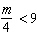a.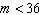;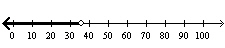c.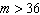;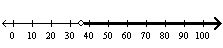b.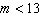;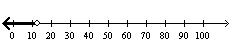d.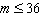;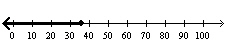2.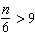a.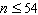;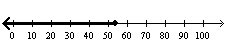c.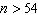;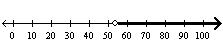b.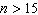;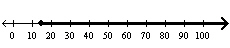d.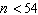;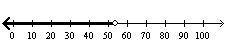3.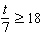a.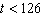;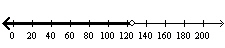c.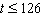;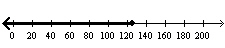b.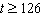;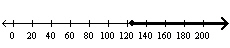d.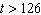;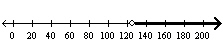4.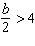a.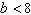;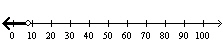c.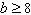;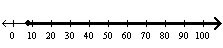b.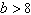;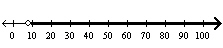d.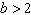;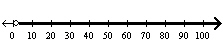5.

The length of a rectangle is 11 feet and its area is less than 88 square feet. Write and solve an inequality to represent the width of the rectangle.
 a.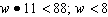ft c.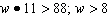ft b.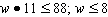ft d.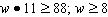ft

Write the word sentence as an inequality. Then solve the inequality.

6.

255 is no less than 15 times a number w.
 a.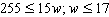c.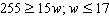b.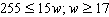d.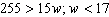Graph the numbers that are solutions to both inequalities.

7.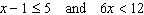a.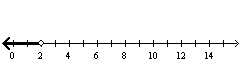c.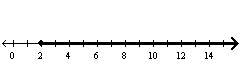b.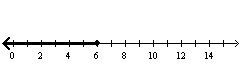d.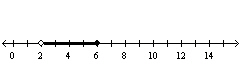Solve the inequality. Graph the solution.

8.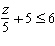a.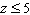;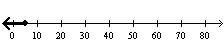c.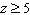;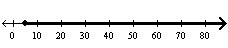b.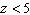;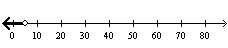d.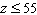;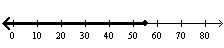9.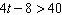a.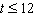;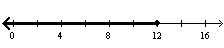c.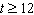;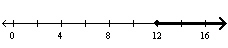b.;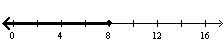d.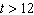;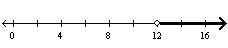10.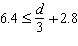a.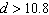;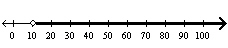c.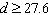;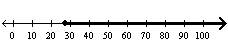b.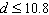;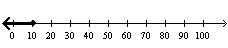d.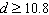;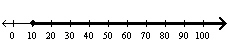The area of the figure is at least 400 square meters.  Write and solve a two-step inequality to represent the possible values of x.  Use 3.14 for p.

1.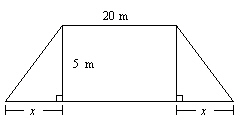2.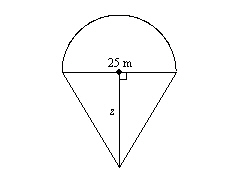3.

The sixth grade goal is to collect at least 300 pledges in the Recycling Pledge Card Contest in your school. Your class has 23 students. The other classes have already completed their collections with a total of 185 pledges. Write and solve an inequality to find how many pledges each student in your class needs for your grade to reach its goal.